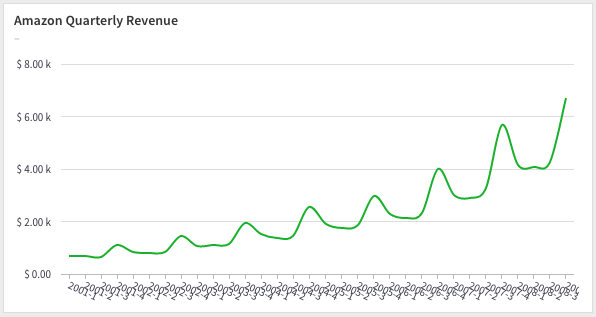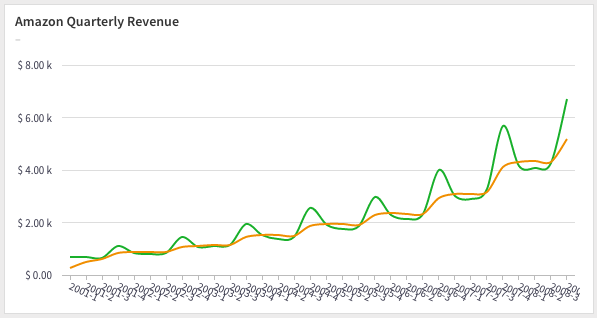# Calculating Weighted Moving Average in SQL

A weighted moving average is a moving average where the previous values within the sliding widnow are given different weights. This is usually done to make recent points more important.

Our previous example of moving average can be thought of a weighted moving average with fractional weights that add up to one. Unfortunately, we cannot build upon that example because we can't make use of window functions.

As before, we chart the quarterly revenues of Amazon for the years 2001 to 2008,We are going to calculate a 4-period moving average. A simple way to compute the weights is to sum up the number of periods,and create fractional weights by dividing the weights by the total.

quarter fraction weight
current 4/10 0.4
current-1 3/10 0.3
current-2 2/10 0.2
current-3 1/10 0.1

You can pick what ever weights you want according to you needs, just make sure the weights add up to one.

Our solution will be a three step process.

## 1. SQL row_number to number the rows

We will first number the rows of the table,

select quarter, revenue, row_number() over ()
from amazon_revenue;

quarter revenue row_number
2001-1 700.356 1
2001-2 667.625 2
2001-3 639.281 3
2001-4 1115.171 4
... ... ...

## 2. SQL self-join to create a sliding window

We will join the above query with itself to create a sliding window over the last three periods,

with t as
(select quarter, revenue, row_number() over ()
from amazon_revenue)
select t.quarter, t.row_number as row_number, t2.quarter as quarter_2, t2.row_number as row_number_2
from t
join t t2 on t2.row_number between t.row_number - 3 and t.row_number


which will give you,

quarter row_number quarter_2 row_number_2
2001-1 1 2001-1 1
2001-2 2 2001-1 1
2001-2 2 2001-2 2
2001-3 3 2001-1 1
2001-3 3 2001-2 2
... ... ... ...

## 3. SQL case to use the fractional weights

Now it's just a matter of finding the difference between the row numbers and applying the fractional weights using a SQL CASE statement.

case
when t.row_number - t2.row_number = 0 then 0.4 * t2.revenue
when t.row_number - t2.row_number = 1 then 0.3 * t2.revenue
when t.row_number - t2.row_number = 2 then 0.2 * t2.revenue
when t.row_number - t2.row_number = 3 then 0.1 * t2.revenue
end


We'll have to group by the quarter and sum up the weighted revenue to calculate the fully weighted average. Here's the full query:

with t as
(select quarter, revenue, row_number() over ()
from amazon_revenue)
select t.quarter, avg(t.revenue) as revenue,
sum(case
when t.row_number - t2.row_number = 0 then 0.4 * t2.revenue
when t.row_number - t2.row_number = 1 then 0.3 * t2.revenue
when t.row_number - t2.row_number = 2 then 0.2 * t2.revenue
when t.row_number - t2.row_number = 3 then 0.1 * t2.revenue
end)
from t
join t t2 on t2.row_number between t.row_number - 3 and t.row_number
group by 1
order by 1👋 No fuss, just SQL We are open sourcing everything from the experience working with our agency clients. They spend thousands of dollars to get this level of detailed analysis – which you can now get for free. We send one update every week. Join 400+ data analysts who are leveling up with our recipes. 👊

No spam, ever! Unsubscribe any time. See past emails here.